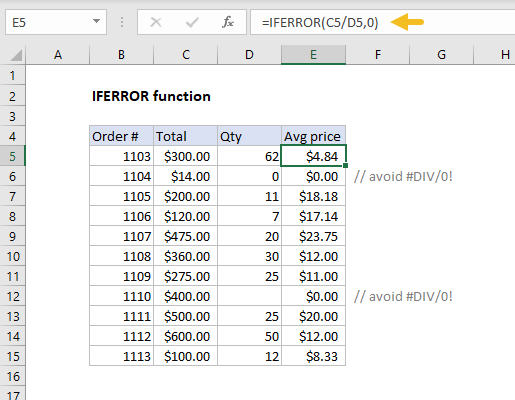Purpose
Trap and handle errors

Return value
The value you specify for error conditions.

Arguments
value - The value, reference, or formula to check for an error.
value_if_error - The value to return if an error is found.

Syntax
=IFERROR(value, value_if_error)

Example #1
In the example shown, the formula in E5 copied down is:
=IFERROR(C5/D5,0)
This formula catches the #DIV/0! error that occurs when Qty is empty or zero, and replaces it with zero.

Example #2
For example, if A1 contains 10, B1 is blank, and C1 contains the formula =A1/B1, the following formula will catch the #DIV/0! error that results from dividing A1 by B1:

=IFERROR (A1/B1,"Please enter a value in B1")
As long as B1 is empty, C1 will display the message "Please enter a value in B1" if B1 is blank or zero. When a number is entered in B1, the formula will return the result of A1/B1.

Example #3
You can also use the IFERROR function to catch the #N/A error thrown by VLOOKUP when a lookup value isn't found. The syntax looks like this: